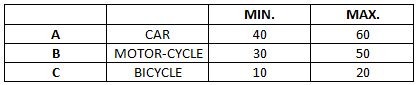### CAT 1997 Question Paper Question 31

Instructions

A certain race is made up of three stretches: A, B and C, each 2 km long, and to be covered by a certain mode of transport. The following table gives these modes of transport for the stretches, and the minimum and maximum possible speeds (in km/hr) over these stretches. The speed over a particular stretch is assumed to be constant. The previous record for the race is 10 min.Question 31

# Anshuman travels at minimum speed by car over A and completes stretch B at the fastest speed. At what speed should he cover stretch C in order to break the previous record?

Solution

Anshuman travels at a minimum speed by car over A, so the time taken = $$\frac{2}{40}$$*60= 3 min
Anshuman travels stretch B at the fastest speed, so the time taken = $$\frac{2}{50}$$*60= 12/5 min
So in order to break the record, he has to cover the three stretches in less than 10 min
10-(3+$$\frac{12}{5}$$) = $$\frac{23}{5}$$
So the time taken to cover stretch C should be less than $$\frac{23}{5}$$ min.
Let x kmph be the speed at which he covers stretch C
$$\frac{2}{x}$$*60 < $$\frac{23}{5}$$
x > 26.08 kmph but the maximum speed at which he can cover stretch C is 20 kmph.
Hence it is not possible for him to break his previous record.